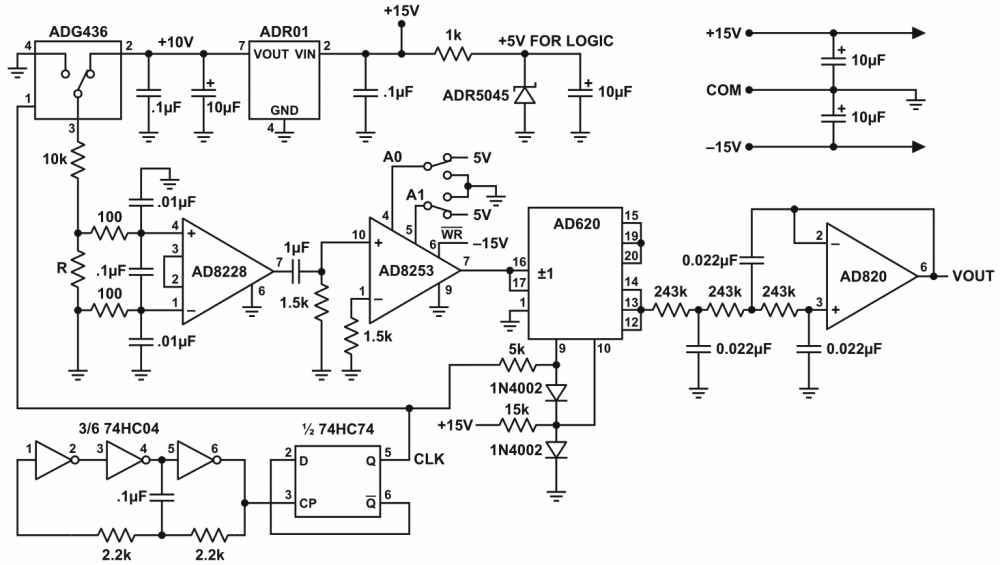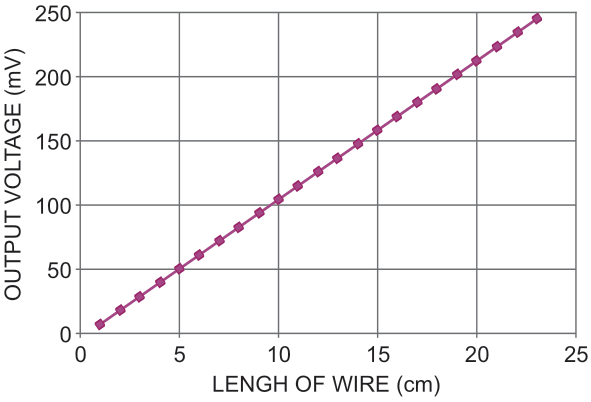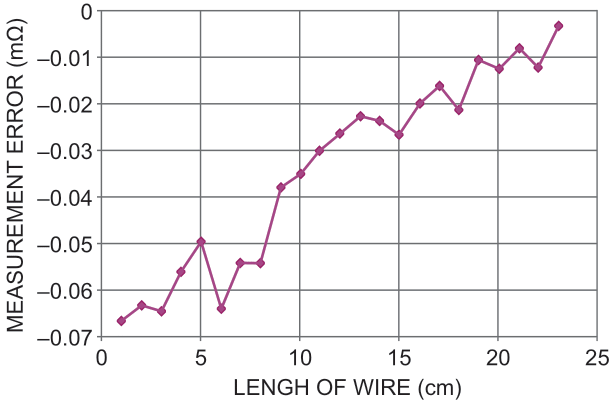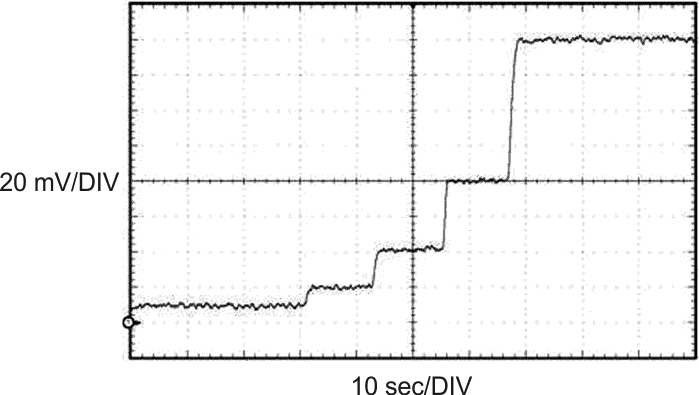# Complete, low-cost, software programmable ohmmeter measures micro-ohms

,

Numerous applications require the measurement of very low resistances, including but not limited to fuse integrity analysis, relay characterization, and superconductor evaluation. There is a wide range of commercially available equipment designed for this task, but these units are prohibitively expensive and cannot be practically integrated into many applications outside the laboratory. A common low-cost and compact method of measuring resistance is to inject a known dc current into the unknown resistor, measure the resulting voltage and calculate the resistance using Ohm’s law. Unfortunately, for very low resistances, the current required to generate a voltage sufficiently distinguishable from the surrounding noise becomes impractically large.Figure 1. The AD8253 is configured in transparent gain mode, allowing the gain to be manually set by the user according to Table 1.

Table 1. Logic levels for AD8253 transparent
 /WR A1 A0 GAIN –VS LOW LOW 1 –VS LOW HIGH 10 –VS HIGH LOW 100 –VS HIGH HIGH 1000

The system is completely self-contained, and the user only needs to provide a ±15 V supply. A 1 kHz oscillator, constructed from common low-cost digital ICs, creates the CLK signal that controls the excitation and demodulation sections of the system, where the 5 V supply for these ICs is obtained from the 15 V rail using an ADR5045 shunt regulator.Figure 2. The output voltage of the system for various lengths of 18 AWG copper wire has a resistance of 213.58 μΩ/cm, as measured in the laboratory

The output voltage of the system is linear over the entire range as can be seen in Figure 2.Figure 3. The measurement error of the system, where the noise in the curve is due to the human error in placing the measurement leads, and the slope of the curve is due to a slight system gain error.

Figure 3 illustrates the measurement error of the system. The linearity of the system is further demonstrated by Figure 4.Figure 4. The linearity of the system is demonstrated by the output voltage of the system as the measurement leads are placed across 1, 2, 4, 8, and 16 cm lengths of wire.

EDN